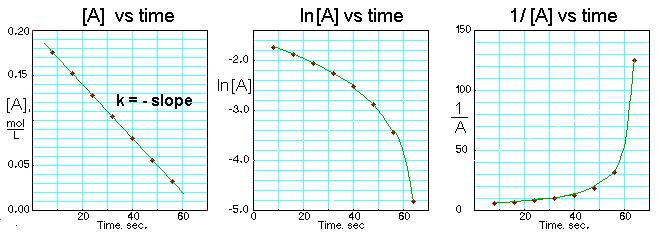Rate Laws from Graphs of Concentration Versus Time (Integrated Rate Laws)

In order to determine the rate law for a reaction from a set of data consisting of concentration (or the values of some function of concentration) versus time, make three graphs.

• [A] versus t (linear for a zero order reaction)
• ln [A] versus t (linear for a 1st order reaction)
• 1 / [A] versus t (linear for a 2nd order reaction)

The graph that is linear indicates the order of the reaction with respect to A. Then, you can choose the correct rate equation:

 For a zero order reaction, rate = k (k = - slope of line) For a 1st order reaction, rate = k[A] (k = - slope of line) For a 2nd order reaction, rate = k[A]2 (k = slope of line)

Examples

For a zero order reaction, as shown in the following figure, the plot of [A] versus time is a straight line with k = - slope of the line. Other graphs are curved for a zero order reaction.For a first order reaction, as shown in the following figure, the plot of the logrithm of [A] versus time is a straight line with k = - slope of the line. Other graphs are curved for a first order reaction.For a second order reaction, as shown in the following figure, the plot of 1/[A] versus time is a straight line with k = slope of the line. Other graphs are curved for a second order reaction.Top